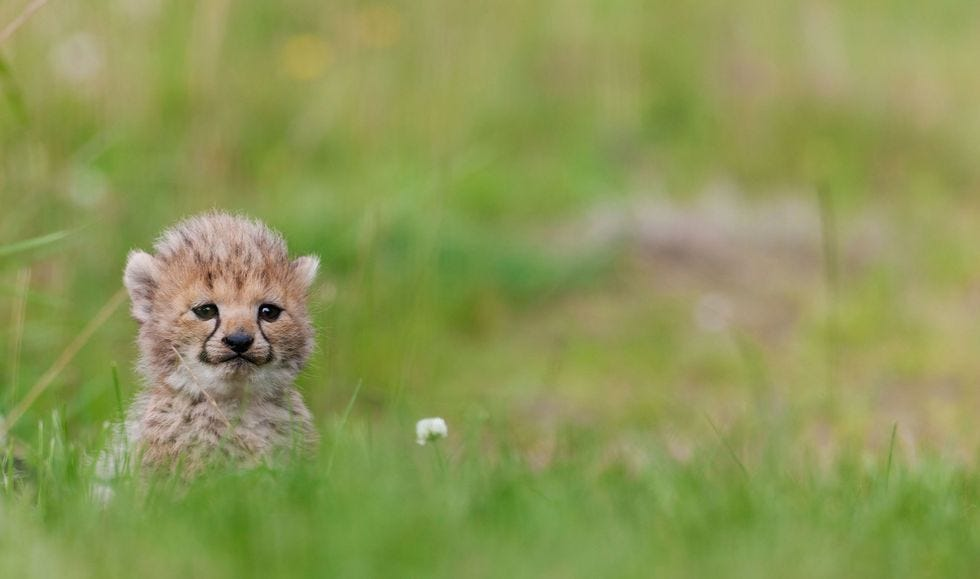# Fixing Small Photos with Deep Learning`import torchimport torch.nn as nnimport torch.nn.functional as Fimport torch.nn.init as init# Main networkclass Net(nn.Module):    def __init__(self, upscale_factor):        super(Net, self).__init__()        self.relu = nn.ReLU()        self.conv1 = nn.Conv2d(1, 64, (5,5), (1,1), (2,2))        self.conv2 = nn.Conv2d(64, 64, (3,3), (1,1), (1,1))        self.conv3 = nn.Conv2d(64, 32, (3,3), (1,1), (1,1))        self.conv4 = nn.Conv2d(32, upscale_factor**2, (3,3), (1,1), (1,1))        self.pixel_shuffle = nn.PixelShuffle(upscale_factor)        self._initialize_weights()    def forward(self, x):        x = self.relu(self.conv1(x))        x = self.relu(self.conv2(x))        x = self.relu(self.conv3(x))        x = self.pixel_shuffle(self.conv4(x))        return x    def _initialize_weights(self):        init.orthogonal_(self.conv1.weight, init.calculate_gain('relu'))        init.orthogonal_(self.conv2.weight,init.calculate_gain('relu'))        init.orthogonal_(self.conv3.weight,init.calculate_gain('relu'))        init.orthogonal_(self.conv4.weight)`